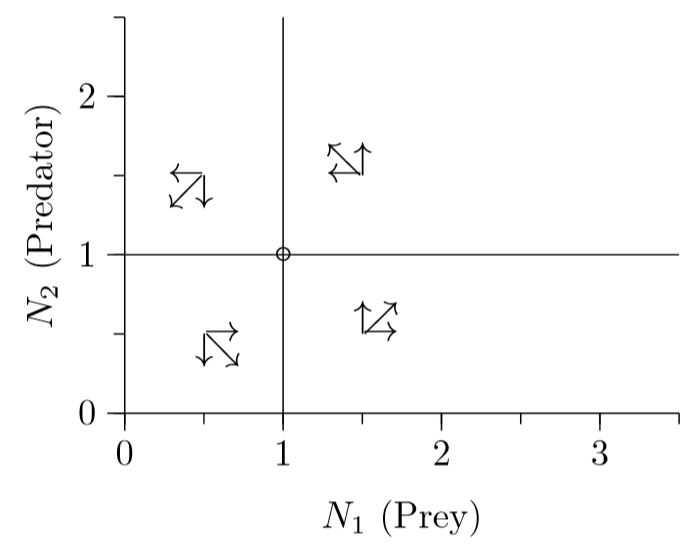# 12.4: Phase Space

$$\newcommand{\vecs}{\overset { \rightharpoonup} {\mathbf{#1}} }$$ $$\newcommand{\vecd}{\overset{-\!-\!\rightharpoonup}{\vphantom{a}\smash {#1}}}$$$$\newcommand{\id}{\mathrm{id}}$$ $$\newcommand{\Span}{\mathrm{span}}$$ $$\newcommand{\kernel}{\mathrm{null}\,}$$ $$\newcommand{\range}{\mathrm{range}\,}$$ $$\newcommand{\RealPart}{\mathrm{Re}}$$ $$\newcommand{\ImaginaryPart}{\mathrm{Im}}$$ $$\newcommand{\Argument}{\mathrm{Arg}}$$ $$\newcommand{\norm}{\| #1 \|}$$ $$\newcommand{\inner}{\langle #1, #2 \rangle}$$ $$\newcommand{\Span}{\mathrm{span}}$$ $$\newcommand{\id}{\mathrm{id}}$$ $$\newcommand{\Span}{\mathrm{span}}$$ $$\newcommand{\kernel}{\mathrm{null}\,}$$ $$\newcommand{\range}{\mathrm{range}\,}$$ $$\newcommand{\RealPart}{\mathrm{Re}}$$ $$\newcommand{\ImaginaryPart}{\mathrm{Im}}$$ $$\newcommand{\Argument}{\mathrm{Arg}}$$ $$\newcommand{\norm}{\| #1 \|}$$ $$\newcommand{\inner}{\langle #1, #2 \rangle}$$ $$\newcommand{\Span}{\mathrm{span}}$$

The cycling can be understood better in phase space, where the densities of the two species are represented as two-dimensional points.Figure $$\PageIndex{1}$$. Predator–prey phase space. The circle marks an equilibrium where growth of both predator and prey stops. The plus sign marks a population value of N1 = 1.5, N2 = 0.5.

For example, as explained in Chapter 10, if the prey population is 1.5 and the predator population is 0.5, the population will be 1.5 units to the right on the horizontal axis and 0.5 units up on the vertical axis, at the location of the blue plus sign in the graph.

Where does the predator population cease to grow? In the equation

$\frac{1}{N_2}\frac{dN_2}{dt}\,=\,r_2\,+\,s_{2,1}N_1$

it ceases to grow where $$0\,=\,r_2\,+\,s_{2,1}N_1$$, or $$N_1\,=\frac{-r_2}{s_{2,1}}$$. With $$r_2$$ = −0.5 and $$s_{2,1}$$ = 0.5, this is a vertical line—the predator isocline—at N1 = 1, as in Figure $$\PageIndex{2}$$.Figure $$\PageIndex{2}$$. The predator isocline, at which the predator population ceases to grow.

To the left of the isocline, prey are sparse and predators decline, as indicated by the downward arrows. To the right of the isocline, in contrast, prey are abundant and predators can increase, as indicated by the upward arrows.

Likewise, where does the prey population cease to grow? In the equation

$\frac{1}{N_1}\frac{dN_1}{dt}\,=\,r_1\,+\,s_{1,2}N_2$

it ceases to grow where 0 = $$r_1\,+\,s_{1,2}N_2$$, which means $$N_2\,=\,\frac{-r_1}{s_{1,2}}$$. With $$r_1$$= 1 and $$s_{1,2}$$ = −1, this is a horizontal line—the prey isocline—at N2 = 1.Figure $$\PageIndex{3}$$. The prey isocline, at which the prey population ceases to grow.

Below the isocline, predators are sparse so prey can increase, as indicated by the arrows pointing right. Above the isocline, in contrast, predators are abundant and prey decreases, as indicated by the arrows pointing left. Putting Figures $$\PageIndex{2}$$ and $$\PageIndex{3}$$ together gives Figure $$\PageIndex{4}$$, which shows rotation in the combined arrows.Figure $$\PageIndex{4}$$. Both isoclines, with both sets of arrows combined, showing the cycling.

Here the rotation can be deduced by thinking about the dynamics of predator and prey. The rotation is corroborated by using Table 10.2.1 to calculate the eigenvalues. The eigenvalues of the interior equilibrium turn out to be 0±0.707$$i$$, a number with both real and imaginary parts. The existence of an imaginary part, ±0.707$$i$$, implies cycling. The real part, 0, means that eigenvalues alone cannot determine the stability—it could be stable, unstable, or neutral. In fact, for this particular case with no self-limitation, deeper mathematical examination shows that the stability is neutral. The dynamics will rotate indefinitely, maintaining whatever cycle it started on.

Taking all the data from Figure 12.3.2 and plotting N1 versus N2 gives Figure $$\PageIndex{5}$$. The process starts at day 0 with N1 = N2 = 2. One day later, prey have dropped to N1 ≈ 0.5 and predators have increased to N2 ≈ 2.2, marked by the red numeral 1 on the cycle. (By the symbol ‘≈’, we mean “approximately equal to.”) Two days later, prey have dropped to N1 ≈ 0.2 and predators have dropped to N2 ≈ 1.0, marked by the numeral 3. With predators at relatively low levels, prey then start to increase and, four days later, have reached N1 ≈ 1.0, while predators have dropped further to N2 ≈ 0.3, marked by the numeral 7. Two days later, prey have increased to N1 ≈ 3.0 and predators have increased to N2 ≈ 1.0, marked by the numeral 9. Finally, one day later the cycle begins to repeat, as marked with the numeral 10. This is another way of showing the cycling of Figure 12.3.2.Figure $$\PageIndex{6}$$. The ﬂow across the phase space.
In Figure $$\PageIndex{6}$$—a ﬂow diagram, the entire phase space can be filled with arrows to show how cycling proceeds everywhere. The path of Figure 12.3.2, displayed in Figure $$\PageIndex{5}$$, is overlayed in blue.